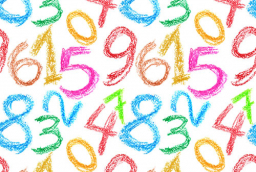# Find two digits

Find the possible values of A and B if the 6 digit number 2A16B6 is divisible by 4 and 9. Result write as composed number.

x1 =  201636
x2 =  221616
x3 =  231696
x4 =  251676
x5 =  271656
x6 =  291636

### Step-by-step explanation:Did you find an error or inaccuracy? Feel free to write us. Thank you!

Tips for related online calculators